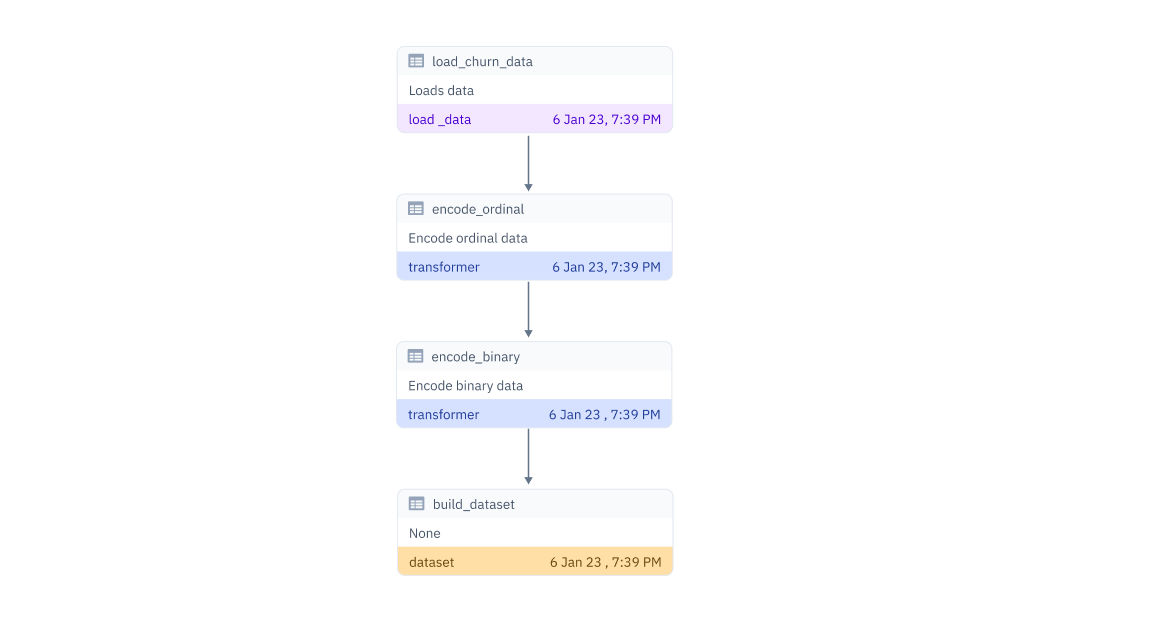Lineage is a crucial element of any data pipeline that enables the tracking of the data flow from its origin to the end goal, which includes all the intermediate processes and transformations. In the context of PureML, lineage involves capturing the provenance of data and transformations applied to produce a final dataset.

### ​Basic Pipeline

A standard data pipeline contains loading data and applying transformations to convert it into a dataset. PureML provides the following decorators to register these components of the pipeline

@load_data is used to decorate a function that is used to load data into the code. It should return the loaded dataframe.

@transformer is used to decorate any function that applies transformations on the data. It should return the loaded dataframe.

@dataset is used to decorate the function that amalgamates all the steps in the data pipeline to generate the dataset. It should return the loaded dataframe.

The following example demonstrates how to create a data pipeline using PureML decorators that capture the lineage of the data transformations

### ​Example

from sklearn.preprocessing import OrdinalEncoder
import pandas as pd
from pureml.decorators import dataset, transformer, load_data

'''
'''

return df

@transformer()
def encode_ordinal(df):
'''
Encode ordinal data
'''
col_ord = ['state', 'phone number']
df_ord = df[col_ord]
feat = OrdinalEncoder().fit_transform(df_ord)

df[col_ord] = feat

return df

@transformer()
def encode_binary(df):
'''
Encode binary data
'''
df['voice mail plan'] = df['voice mail plan'].map({'yes':1, 'no':0})
df['international plan'] = df['international plan'].map({'yes':1, 'no':0})
df['churn'] = df['churn'].map({True:1, False:0})

return df

@dataset('telecom_churn:dev')
def build_dataset():
'''
None
'''